## A man can lift a mass of 200kg onThe surface of the earth. what is the amount of mass he can lift on the surface of the moon?

Question

A man can lift a mass of 200kg onThe surface of the earth. what is the amount of mass he can lift on the surface of the moon?

in progress 0
5 months 2021-09-05T16:35:24+00:00 1 Answers 23 views 0

## Answers ( )

1,211.1 kg.

Explanation:

the force of gravity is less on the moon than on earth, so if the man can lift 200kg on earth, he could lift a greater amount on the moon because there is less resistance from gravity.

To know the amount of mass he can lift on the moon, we first need to know the amount of weight that is equivalent to those 200kg here on earth. This because the weight of the object is equal to the force that must be applied to lift it, and that force is applied by the man and it will be the same here and on the moon.

We calculate weight using the formula: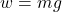where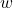is the weight of the object (the force with which the earth attracts the object)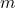is the mass and g the acceleration of gravity.

so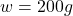for earth the acceleration due to gravity is: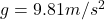thus: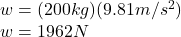now we use this value to calculate the mass he can lift on the moon, since for the moon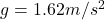.

we use the same equation, w =mg substituting w = 1962N and: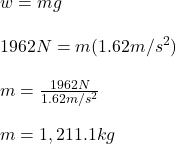he can lift 1,211.1 kg.

You can also find the result using the approximate value of the acceleration of gravity on the moon as g/6, where g is the acceleration on earth.